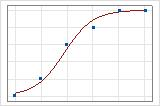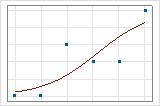# Model summary table for Fit Poisson Model

Find definitions and interpretation guidance for every statistic in the Model summary table.

## Deviance R-Sq

Deviance R2 is usually considered the proportion of the total deviance in the response variable that the model explains.

### Interpretation

The higher the deviance R2, the better the model fits your data. Deviance R2 is always between 0% and 100%.

Deviance R2 always increases when you add additional terms to a model. For example, the best 5-term model will always have an R2 that is at least as high as the best 4-term model. Therefore, deviance R2 is most useful when you compare models of the same size.

Goodness-of-fit statistics are just one measure of how well the model fits the data. Even when a model has a desirable value, you should check the residual plots and goodness-of-fit tests to assess how well a model fits the data.

You can use a fitted line plot to graphically illustrate different deviance R2 values. The first plot illustrates a model that explains approximately 96% of the deviance in the response. The second plot illustrates a model that explains about 60% of the deviance in the response. The more deviance that a model explains, the closer the data points fall to the curve. Theoretically, if a model could explain 100% of the deviance, the fitted values would always equal the observed values and all of the data points would fall on the curve.The data arrangement affects the deviance R2 value. The deviance R2 is usually higher for data with multiple trials per row than for data with a single trial per row. Deviance R2 values are comparable only between models that use the same data format. For more information, go to How data formats affect goodness-of-fit in binary logistic regression.

Adjusted deviance R2 is the proportion of deviance in the response that is explained by the model, adjusted for the number of predictors in the model relative to the number of observations.

### Interpretation

Use adjusted deviance R2 to compare models that have different numbers of terms. Deviance R2 always increases when you add a term to the model. The adjusted deviance R2 value incorporates the number of terms in the model to help you choose the correct model.

For example, you work for a potato chip company that examines factors that affect crumbled potato chips. You receive the following results as you add predictors:
Step % Potato Cooling rate Cooking temp Deviance R2 Adjusted Deviance R2 P-value
1 X     52% 51% 0.000
2 X X   63% 62% 0.000
3 X X X 65% 62% 0.000

The first step yields a statistically significant regression model. The second step, which adds cooling rate to the model, increases the adjusted deviance R2, which indicates that cooling rate improves the model. The third step, which adds cooking temperature to the model, increases the deviance R2 but not the adjusted deviance R2. These results indicate that cooking temperature does not improve the model. Based on these results, you consider removing cooking temperature from the model.

The data arrangement affects the adjusted deviance R2 value. For the same data, the adjusted deviance R2 is usually higher for data with multiple trials per row than for data with a single trial per row. Use the adjusted deviance R2 only to compare the fit of models that have the same data format. For more information, go to How data formats affect goodness-of-fit in binary logistic regression.

## Test Deviance R-Sq

Test deviance R2 is usually considered the proportion of the total deviance in the response variable of the test data set that the model explains.

### Interpretation

Use test deviance R2 to determine how well your model fits new data. Models that have larger test deviance R2 values tend to perform better on new data. You can use test deviance R2 to compare the performance of different models.

A test deviance R2 that is substantially less than deviance R2 may indicate that the model is over-fit. An over-fit model occurs when you add terms for effects that are not important in the population. The model becomes tailored to the training data and, therefore, may not be useful for making predictions about the population.

For example, an analyst at a financial consulting company develops a model to predict future market conditions. The model looks promising because it has an R2 of 87%. However, the test deviance R2 is 52%, which indicates that the model may be over-fit.

A high test deviance R2 value by itself does not indicate that the model meets the model assumptions. You should check the residual plots to verify the assumptions.

## K-Fold Deviance R-sq

K-fold deviance R2 is usually considered the proportion of the total deviance in the response variable of the validation data that the model explains.

### Interpretation

Use k-fold deviance R2 to determine how well your model fits new data. Models that have larger k-fold deviance R2 values tend to perform better on new data. You can use k-fold deviance R2 values to compare the performance of different models.

A k-fold deviance R2 that is substantially less than deviance R2 can indicate that the model is over-fit. An over-fit model occurs when you add terms for effects that are not important in the population. The model becomes tailored to the training data set and, therefore, might not be useful for making predictions about the population.

For example, an analyst at a financial consulting company develops a model to predict future market conditions. The model looks promising because it has a deviance R2 of 87%. However, the k-fold deviance R2 is 52%, which indicates that the model might be over-fit.

A high k-fold deviance R2 value by itself does not indicate that the model meets the model assumptions. You should check the residual plots to verify the assumptions.

## AIC, AICc and BIC

The Akaike's Information Criterion (AIC), corrected Akaike’s Information Criterion (AICc), and the Bayesian Information Criterion (BIC) are measures of the relative quality of a model that account for fit and the number of terms in the model.

### Interpretation

Use AIC, AICc and BIC to compare different models. Smaller values are desirable. However, the model with the least value for a set of predictors does not necessarily fit the data well. Also use tests and plots to assess how well the model fits the data.
AICc and AIC
When the sample size is small relative to the parameters in the model, AICc performs better than AIC. AICc performs better because with relatively small sample sizes, AIC tends to be small for models with too many parameters. Usually, the two statistics give similar results when the sample size is large enough relative to the parameters in the model.
AICc and BIC
Both AICc and BIC assess the likelihood of the model and then apply a penalty for adding terms to the model. The penalty reduces the tendency to overfit the model to the sample data. This reduction can yield a model that performs better in general.
As a general guideline, when the number of parameters is small relative to the sample size, BIC has a larger penalty for the addition of each parameter than AICc. In these cases, the model that minimizes BIC tends to be smaller than the model that minimizes AICc.
In some common cases, such as screening designs, the number of parameters is usually large relative to the sample size. In these cases, the model that minimizes AICc tends to be smaller than the model that minimizes BIC. For example, for a 13-run definitive screening design, the model that minimizes AICc will tend to be smaller than the model that minimizes BIC among the set of models with 6 or more parameters.